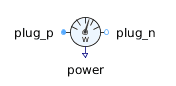Leakage Around Poles - MapleSim Help

Leakage Around Poles

Leakage flux tube around cylindrical or prismatic polesDescription The Leakage around Poles component models the leakage magnetic flux around a prismatic air-gap between two poles. It models the radius of the leakage field as a constant parameter, independent of the length of the air-gap.Equations ${F}_{m}=\frac{1}{2}{V}_{m}^{2}\frac{{\mathrm{dG}}_{m}}{\mathrm{dx}}$ ${G}_{m}={\mathrm{\mu }}_{0}\frac{w}{\mathrm{\pi }}\mathrm{log}\left(1+\mathrm{\pi }\frac{r}{\ell }\right)$ ${R}_{m}=\frac{1}{{G}_{m}}$ $\mathrm{\Phi }={\mathrm{\Phi }}_{p}=-{\mathrm{\Phi }}_{n}$ ${V}_{m}={V}_{{m}_{p}}-{V}_{{m}_{n}}={R}_{m}\mathrm{\Phi }$ $\frac{{\mathrm{dG}}_{m}}{\mathrm{dx}}=\frac{-{\mathrm{\mu }}_{0}wr}{{\ell }^{2}\left(1+\mathrm{\pi }\frac{r}{\ell }\right)}\frac{d\ell }{\mathrm{dx}}$ ${f}_{\mathrm{flange}}=-{F}_{m}$Variables

 Name Units Description Modelica ID ${V}_{m}$ $A$ Magnetic potential difference between both ports V_m $\mathrm{\Phi }$ $\mathrm{Wb}$ Magnetic flux from port_p to port_n Phi ${F}_{m}$ $N$ Reluctance force F_m $s$ $m$ Distance between flange and support s ${R}_{m}$ ${H}^{-1}$ Magnetic reluctance R_m ${G}_{m}$ $H$ Magnetic permeance G_m $\frac{{\mathrm{dG}}_{m}}{\mathrm{dx}}$ $\frac{H}{m}$ Derivative of permeance with respect to armature position dGmBydx $\ell$ $m$ Axial length (in direction of flux) lConnections

 Name Description Modelica ID ${\mathrm{port}}_{p}$ Positive magnetic port port_p ${\mathrm{port}}_{n}$ Negative magnetic port port_n $\mathrm{flange}$ Generated reluctance force at armature position flange $\mathrm{support}$ supportParameters

 Name Default Units Description Modelica ID ${\mathrm{\mu }}_{r}$ $1$ $1$ Relative magnetic permeability mu_r $\frac{d\ell }{\mathrm{dx}}$ $1$ $1$ Derivative of flux tube's varying dimension with respect to armature position; set to +1 or -1 dl/dx $\mathrm{\omega }$ $0.1$ $m$ Width orthogonal to flux; mean circumference of flux tube in case of cylindrical poles w $r$ $0.01$ $m$ Radius of leakage field r Use Support $\mathrm{false}$ True (checked) enables the support flange useSupportModelica Standard Library The component described in this topic is from the Modelica Standard Library. To view the original documentation, which includes author and copyright information, click here.